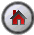SHAZAM Dummy Variables

## Dummy Variables

Dummy variables are very useful for capturing a variety of qualitative effects. They typically have the value 0 or 1 and so possibly a better name is "binary variable". They can be included as explanatory variables in the regression equation and the estimated coefficients and standard errors can be used in hypothesis testing.

Dummy variables that measure individual attributes may be coded in the data file and these can be assigned variable names and loaded into SHAZAM with the `READ` command. Alternatively, it may be convenient to create the required dummy variables with SHAZAM commands.

#### Examples

Note: When dummy variables are used as dependent variables then analysis with the probit or logit model is required.[SHAZAM Guide home]

### Modelling structural change

Consider measuring the impact of seat belt legislation on traffic fatalities. Monthly data is available for 120 observations on traffic fatalities (`D`) and auto fuel consumption (`C`). Suppose that seat belt legislation became effective at observation 80. To estimate the impact of this legislation define a dummy variable as follows:

```            B = 0      for observations 1 to 79
B = 1      for observations 80 to 120
```

The following SHAZAM commands create the dummy variable and run the regression.

```SAMPLE 1 120
* Create the dummy variable
GENR B=0
SAMPLE 80 120
GENR B=1
*
SAMPLE 1 120
* List the data and check that it is set up correctly.
PRINT D C B
* Get the OLS estimation results
OLS D C B
STOP
```

Note that the command `GENR B=0` first initializes the dummy variable to all zeroes. Then the `SAMPLE` command is used to set the sample to the period of structural change and the dummy variable is then set to 1 for this period. The dummy variable is now created and the OLS estimation is then run over the entire sample period of the data.

In SHAZAM there is often more than one way of doing things. An equivalent way of creating the dummy variable is illustrated in the next list of commands.

```SAMPLE 1 120
The dummy variable is created in a single command by using the `DUM` and `TIME` functions on the `GENR` command. The `TIME(0)` function creates an observation index with values 1,2,3,. . . . The `DUM` function is set to 1 when the argument is > 0 (that is, positive values) and is set to 0 otherwise. In this example the argument of the `DUM` function is `TIME(0)-79`. This will have positive values for observation numbers that are greater than or equal to 80.